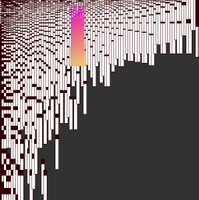# Processing point of view

I have tried processing by entering a few lines of script into the processing window.

processing en essais

For instance I wrote the following script:
size(600, 600);
background(50,50,50);
for(int x=0; x<600; x="x+7){" y="0;" r =" (int)(40*(log(x)));" g =" (int)(30*(log(y)));" b =" (int)(r*g" gr =" (r"> 128){
stroke(r, g, b);
rect(r,g, 1, 8);
}
else{
stroke(50,0,0);
rect(x,y, 10, 91);
}

}
}

and processing has generated the following graphic:Rendez-vous creation
has selected a few images I made with processing.

In digital graphics

#### 1 comment:Anonymous said...

It reminds me that Processing is an interesting tool to try. Thank you Cati ;-)